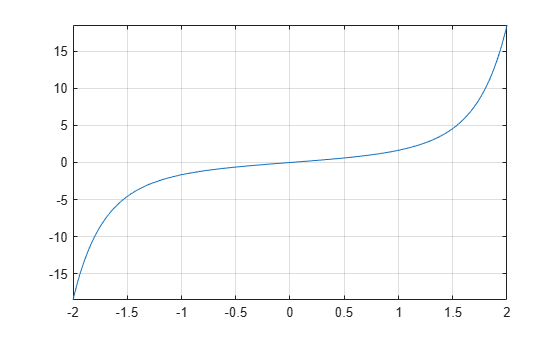# erfi

Imaginary error function

## Syntax

``erfi(x)``

## Description

example

````erfi(x)` returns the imaginary error function of `x`. If `x` is a vector or a matrix, `erfi(x)` returns the imaginary error function of each element of `x`.```

## Examples

### Imaginary Error Function for Floating-Point and Symbolic Numbers

Depending on its arguments, `erfi` can return floating-point or exact symbolic results.

Compute the imaginary error function for these numbers. Because these numbers are not symbolic objects, you get floating-point results.

`s = [erfi(1/2), erfi(1.41), erfi(sqrt(2))]`
```s = 0.6150 3.7382 3.7731```

Compute the imaginary error function for the same numbers converted to symbolic objects. For most symbolic (exact) numbers, `erfi` returns unresolved symbolic calls.

`s = [erfi(sym(1/2)), erfi(sym(1.41)), erfi(sqrt(sym(2)))]`
```s = [ erfi(1/2), erfi(141/100), erfi(2^(1/2))]```

Use `vpa` to approximate this result with the 10-digit accuracy:

`vpa(s, 10)`
```ans = [ 0.6149520947, 3.738199581, 3.773122512]```

### Imaginary Error Function for Variables and Expressions

Compute the imaginary error function for `x` and ```sin(x) + x*exp(x)```. For most symbolic variables and expressions, `erfi` returns unresolved symbolic calls.

```syms x f = sin(x) + x*exp(x); erfi(x) erfi(f)```
```ans = erfi(x) ans = erfi(sin(x) + x*exp(x))```

### Imaginary Error Function for Vectors and Matrices

If the input argument is a vector or a matrix, `erfi` returns the imaginary error function for each element of that vector or matrix.

Compute the imaginary error function for elements of matrix `M` and vector `V`:

```M = sym([0 inf; 1/3 -inf]); V = sym([1; -i*inf]); erfi(M) erfi(V)```
```ans = [ 0, Inf] [ erfi(1/3), -Inf] ans = erfi(1) -1i```

### Special Values of Imaginary Error Function

Compute the imaginary error function for x = 0, x = ∞, and x = –∞. Use `sym` to convert `0` and infinities to symbolic objects. The imaginary error function has special values for these parameters:

`[erfi(sym(0)), erfi(sym(inf)), erfi(sym(-inf))]`
```ans = [ 0, Inf, -Inf]```

Compute the imaginary error function for complex infinities. Use `sym` to convert complex infinities to symbolic objects:

`[erfi(sym(i*inf)), erfi(sym(-i*inf))]`
```ans = [ 1i, -1i]```

### Handling Expressions That Contain Imaginary Error Function

Many functions, such as `diff` and `int`, can handle expressions containing `erfi`.

Compute the first and second derivatives of the imaginary error function:

```syms x diff(erfi(x), x) diff(erfi(x), x, 2)```
```ans = (2*exp(x^2))/pi^(1/2) ans = (4*x*exp(x^2))/pi^(1/2)```

Compute the integrals of these expressions:

```int(erfi(x), x) int(erfi(log(x)), x)```
```ans = x*erfi(x) - exp(x^2)/pi^(1/2) ans = x*erfi(log(x)) - int((2*exp(log(x)^2))/pi^(1/2), x)```

### Plot Imaginary Error Function

Plot the imaginary error function on the interval from -2 to 2.

```syms x fplot(erfi(x),[-2,2]) grid on```## Input Arguments

collapse all

Input, specified as a floating-point or symbolic number, variable, expression, function, vector, or matrix.

collapse all

### Imaginary Error Function

The imaginary error function is defined as:

`$erfi\left(x\right)=-i\text{\hspace{0.17em}}erf\left(ix\right)=\frac{2}{\sqrt{\pi }}\underset{0}{\overset{x}{\int }}{e}^{{t}^{2}}dt$`

## Tips

• `erfi` returns special values for these parameters:

• `erfi(0) = 0`

• `erfi(inf) = inf`

• `erfi(-inf) = -inf`

• `erfi(i*inf) = i`

• `erfi(-i*inf) = -i`